#Function Repository Resource:

# Inline

Insert the values of symbols into an expression before evaluation

Contributed by: Richard Hennigan (Wolfram Research)
 ResourceFunction["Inline"][symbol,expr] inserts the value of symbol into expr. ResourceFunction["Inline"][{sym1,sym2,…},expr] inserts the values of the symbols sym1,sym2,… into expr.

## Examples

### Basic Examples

Inline the value of a symbol into a held expression:

 In:=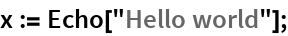In:=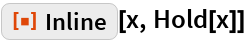Out=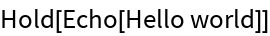### Scope

Retrieve the ResourceFunction:

 In:=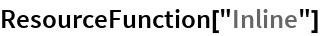Out=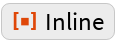Retrieve the ResourceObject:

 In:=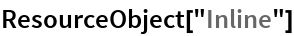Out=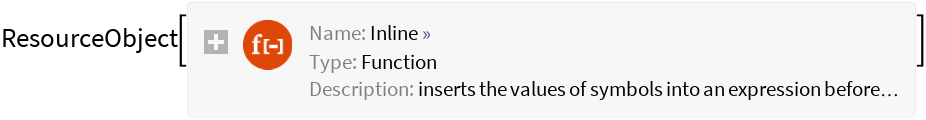Inline multiple symbols:

 In:=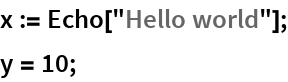In:=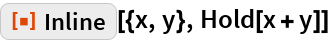Out=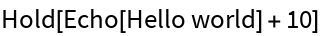### Generalizations and Extensions

For values defined with Set, Inline is functionally similar to using With in order to insert values:

 In:=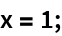In:=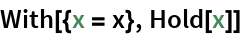Out=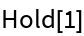In:=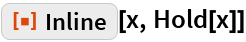Out=Inline also works for delayed values without evaluating the value:

 In:=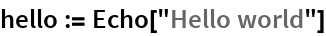In:=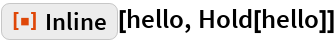Out=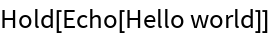In:=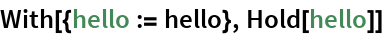Out=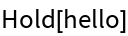Inline a function into an expression:

 In:=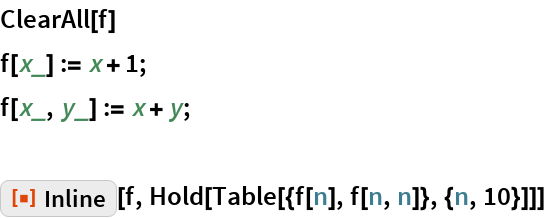Out=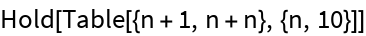### Options

#### MaxIterations

Limit the number of replacements that are made:

 In:=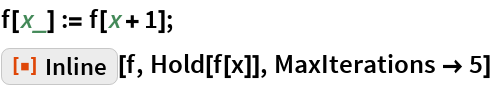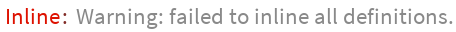Out=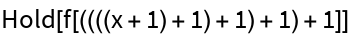### Possible Issues

Patterns are matched using the unevaluated expression:

 In:=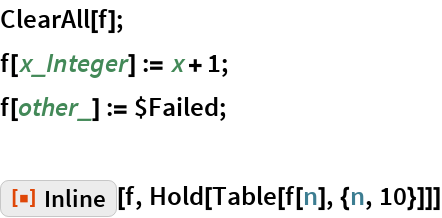Out=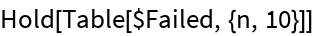Recursive functions usually cannot be completely eliminated:

 In:=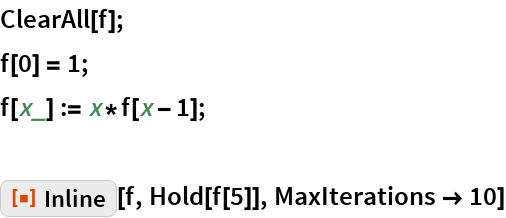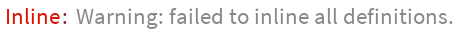Out=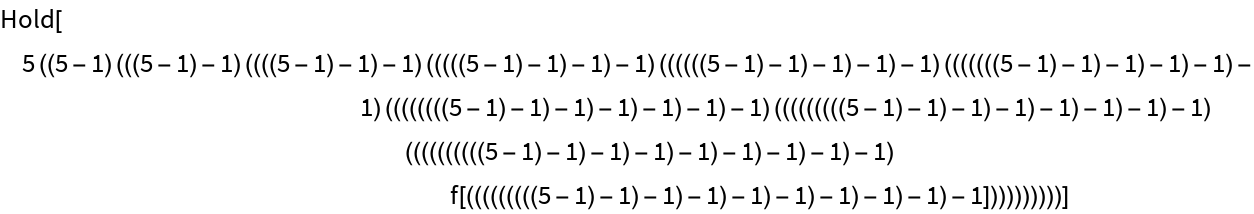## Requirements

Wolfram Language 11.3 (March 2018) or above